# MA.5.AR.1.2Export Print
Solve real-world problems involving the addition, subtraction or multiplication of fractions, including mixed numbers and fractions greater than 1.

### Examples

Shanice had a sleepover and her mom is making French toast in the morning. If her mom had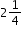loaves of bread and used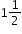loaves for the French toast, how much bread does she have left?

### Clarifications

Clarification 1: Instruction includes the use of visual models and equations to represent the problem.
General Information
Subject Area: Mathematics (B.E.S.T.)
Strand: Algebraic Reasoning
Status: State Board Approved

## Benchmark Instructional Guide

• NA

### Vertical Alignment

Previous Benchmarks

Next Benchmarks

### Purpose and Instructional Strategies

The purpose of this benchmark is to continue the work from grade 4 where students began solving real-world with fractions, and prepares them for grade 6 (MA.6.NSO.2.3) where they will solve real-world fraction problems using all four operations with fractions (MTR.7.1).
• Students need to develop an understanding that when adding or subtracting fractions, the fractions must refer to the same whole.
• During instruction, teachers should provide opportunities for students to practice solving problems using models or drawings to add, subtract or multiply with fractions. Begin with students modeling with whole numbers, have them explain how they used the model or drawing to arrive at the solution, then scaffold using the same methodology using fraction models.
• Models to consider when solving fraction problems should include, but are not limited to, area models (rectangles), linear models (fraction strips/bars and number lines) and set models (counters) (MTR.2.1).
• Please note that it is not expected for students to always find least common multiples or make fractions greater than 1 into mixed numbers, but it is expected that students know and understand equivalent fractions, including naming fractions greater than 1 as mixed numbers to add, subtract or multiply.
• It is important that teachers have students rename the fractions with a common denominator when solving addition and subtraction fraction problems in lieu of the “butterfly” method (or other shortcut/mnemonic) to ensure students build a complete conceptual understanding of what makes solving addition and subtraction of fractions problems true.

### Common Misconceptions or Errors

• When solving real-world problems, students can often confuse contexts that require subtraction and multiplication of fractions.
• For example, “Mark has $\frac{\text{3}}{\text{4}}$ yards of rope and he gives half of the rope to a friend. How much rope does Mark have left?” expects students to find $\frac{\text{1}}{\text{2}}$ of $\frac{\text{3}}{\text{4}}$, or multiply $\frac{\text{1}}{\text{2}}$ × $\frac{\text{3}}{\text{4}}$ to find the product that represents how much is given to the friend. On the other hand, “Mark has $\frac{\text{3}}{\text{4}}$ yards of rope and gives $\frac{\text{1}}{\text{2}}$ yard of rope to a friend. How much rope does Mark have left?” expects students to take $\frac{\text{1}}{\text{2}}$ yard from $\frac{\text{3}}{\text{4}}$ yard, or subtract $\frac{\text{3}}{\text{4}}$$\frac{\text{1}}{\text{2}}$ to find the difference. Encourage students to look for the units in the problem (e.g., $\frac{\text{1}}{\text{2}}$ yard versus $\frac{\text{1}}{\text{2}}$of the whole rope) to determine the appropriate operation.
• Students may believe that multiplication always results in a larger number. Using models when multiplying with fractions will enable students to generalize about multiplication algorithms that are based on conceptual understanding (MTR.5.1).
• Students can have difficulty with word problems when determining which operation to use, and the stress of working with fractions makes this happen more often.
• For example, “Mark has $\frac{\text{3}}{\text{4}}$ yards of rope and he gives a third of the rope to a friend. How much rope does Mark have left?” expects students to first find $\frac{\text{1}}{\text{3}}$ of $\frac{\text{3}}{\text{4}}$, or multiply 1/3 × $\frac{\text{3}}{\text{4}}$, and then to find the difference to find how much Mark has left. On the other hand, "Mark has $\frac{\text{3}}{\text{4}}$ yards of rope and gives $\frac{\text{1}}{\text{3}}$ yard of rope to a friend. How much rope does Mark have left?” only requires finding the difference $\frac{\text{3}}{\text{4}}$$\frac{\text{1}}{\text{3}}$.

### Strategies to Support Tiered Instruction

• Instruction includes opportunities to identify the appropriate operation to use in a real- world problem that requires addition, subtraction, or multiplication of fractions. The teacher guides students to identify the units in the problem for clarification on which operation is appropriate.
• For example, the teacher displays and reads the following two problems:
• “Ganie has $\frac{\text{7}}{\text{8}}$ of a bar of chocolate left and gives half of what she has to her friend Sarah. How much of a whole chocolate bar does she have left?”
• “Ganie has $\frac{\text{7}}{\text{8}}$ of a bar of chocolate left and she gives $\frac{\text{1}}{\text{2}}$ of the original bar of chocolate to her friend Sarah. How much of her chocolate bar does she have left?” (See illustration below)
• The teacher uses questioning and prompting to have students identify what operations must be used to solve each problem. The teacher asks students to share what they notice about each problem (e.g., the similarities and the differences), placing emphasis on the units (e.g., “half of the amount of chocolate that Janie has in the first problem vs. $\frac{\text{1}}{\text{2}}$ of the whole chocolate bar” in the second problem). The teacher guides students to identify that in the first problem, they will need to multiply $\frac{\text{7}}{\text{8}}$ × $\frac{\text{1}}{\text{2}}$ and in the second problem, they will need to subtract $\frac{\text{7}}{\text{8}}$ − $\frac{\text{1}}{\text{2}}$ to solve. Students solve using models.
$\frac{\text{7}}{\text{8}}$×$\frac{\text{1}}{\text{2}}$=$\frac{\text{7}}{\text{16}}$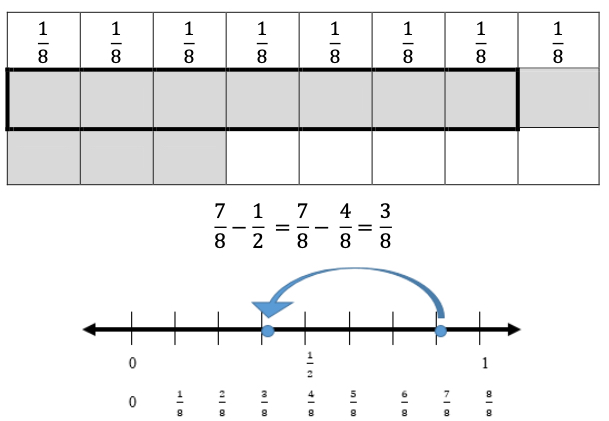• For example, the teacher displays and reads the following problem: "Tia has $\frac{\text{3}}{\text{8}}$ yards of ribbon and she gives half of the ribbon to a friend. How much ribbon does Tia have left?” The teacher uses questioning and prompting to have students identify what operation must be used to solve the problem. The teacher asks students, “Did Tia give half of the ribbon or half a yard of ribbon to her  friend?” Emphasis is placed on the units (e.g., half of the whole ribbon vs. $\frac{\text{1}}{\text{2}}$ yard of ribbon) while guiding students to identify that they will need to multiply $\frac{\text{3}}{\text{8}}$ × $\frac{\text{1}}{\text{2}}$ to solve. Students solve using the area model and counters. The cells with both color counters indicate the numerator in the solution. This is repeated with similar word problems, using frequent guiding questions to support student understanding.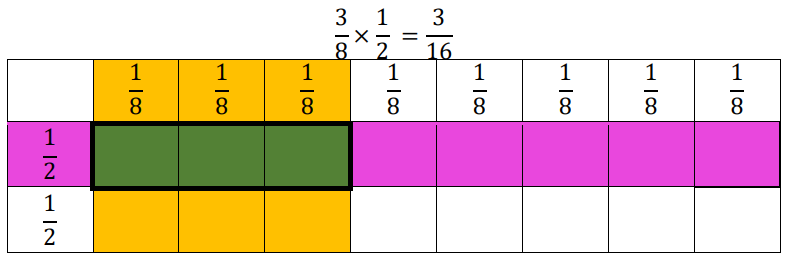• Instruction includes opportunities to use models when solving problems that involve multiplication of fractions to increase understanding that multiplication does not always result in a larger number. The use of models when multiplying with fractions will enable students to generalize about multiplication algorithms that are based on conceptual understanding.
• For example, the teacher displays and reads aloud the following problem: “Rosalind spent $\frac{\text{2}}{\text{3}}$ of an hour helping in the garden. Her sister spent $\frac{\text{1}}{\text{2}}$ the amount of time as Rosalind did helping in the garden. How much time did Rosalind’s sister spend helping in the garden?” Students solve the problem using an area model. The teacher uses questioning to help students draw a model to represent the problem. This is repeated with similar word problems involving multiplication of fractions.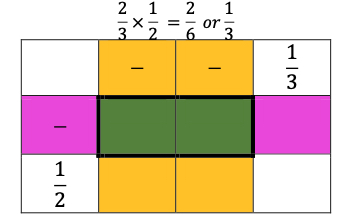• For example, the teacher displays and reads aloud the following problem: "Astrid spent $\frac{\text{7}}{\text{8}}$ of an hour reading her book. Elliot spent $\frac{\text{1}}{\text{3}}$ the amount of time as Astrid did reading. How much time did Elliot spend reading?” Students solve using the area model and counters. The cells with both color counters indicate the numerator in the solution. The teacher uses questioning to help students draw a model to represent the problem. This is repeated with similar word problems involving multiplication of fractions, using frequent guiding questions to support student understanding.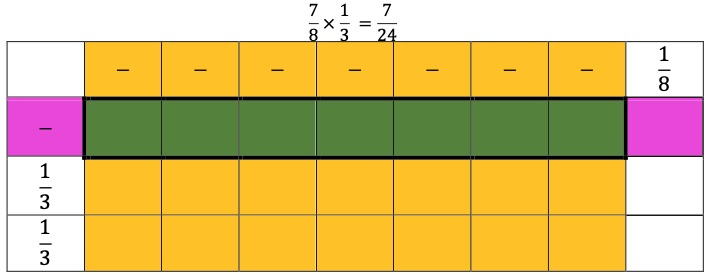Rachel wants to bake her two favorite brownie recipes. One recipe needs 1 $\frac{\text{1}}{\text{2}}$ cups of flour and the other recipe needs $\frac{\text{3}}{\text{4}}$ cups of flour. How much flour does Rachel need to bake her two favorite brownie recipes?

Shawn finished a 100 meter race in $\frac{\text{3}}{\text{8}}$ of one minute. The winner of the race finished in $\frac{\text{1}}{\text{3}}$ of Shawn’s time. How long did it take for the winner of the race to finish?

### Instructional Items

Instructional Item 1

Monica has 2 $\frac{\text{3}}{\text{4}}$ cups of berries. She uses $\frac{\text{5}}{\text{8}}$ cups of berries to make a smoothie. She then uses $\frac{\text{1}}{\text{2}}$ cup for a fruit salad. After she makes her smoothie and fruit salad, how much of the berries will Monica have left?

*The strategies, tasks and items included in the B1G-M are examples and should not be considered comprehensive.

## Related Courses

This benchmark is part of these courses.
5012070: Grade Five Mathematics (Specifically in versions: 2014 - 2015, 2015 - 2022, 2022 and beyond (current))
7712060: Access Mathematics Grade 5 (Specifically in versions: 2014 - 2015, 2015 - 2018, 2018 - 2022, 2022 and beyond (current))
5012065: Grade 4 Accelerated Mathematics (Specifically in versions: 2019 - 2022, 2022 and beyond (current))
5012015: Foundational Skills in Mathematics 3-5 (Specifically in versions: 2019 - 2022, 2022 and beyond (current))

## Related Access Points

Alternate version of this benchmark for students with significant cognitive disabilities.
MA.5.AR.1.AP.2a: Solve one-step real-world problems involving addition and subtraction of mixed numbers and fractions greater than one with like denominators.
MA.5.AR.1.AP.2b: Solve one-step real-world problems involving multiplication of unit fractions.

## Related Resources

Vetted resources educators can use to teach the concepts and skills in this benchmark.

## Formative Assessments

Maris Has a Party:

Students are given a word problem involving fractions with unlike denominators and are asked to estimate the sum, explain their reasoning, and then determine the sum.

Type: Formative Assessment

Sarah’s Hike:

Students are asked to estimate the difference between two fractional lengths and then calculate the difference.

Type: Formative Assessment

Pizza Party:

Students are asked to solve a word problem by finding the product of two fractions.

Type: Formative Assessment

Just Run:

Students are given a word problem involving subtraction of fractions with unlike denominators. Students are asked to determine if a given answer is reasonable, explain their reasoning, and calculate the answer.

Type: Formative Assessment

Half of a Recipe:

Students are asked to solve a word problem by finding the product of a fraction and a mixed number.

Type: Formative Assessment

Candy at the Party:

Students are asked to solve a word problem by finding the product of two mixed numbers.

Type: Formative Assessment

Box Factory:

Students are asked to solve a word problem by finding the product of two fractions.

Type: Formative Assessment

Baking Cakes:

Students are asked to estimate the sum of two mixed numbers and then calculate the sum.

Type: Formative Assessment

## Lesson Plans

Students will help the Supervisor of Elections determine which voter registration locations could be improved to help more citizens get registered to vote. Students will learn about the number of citizens who registered to vote in a general election year compared to the total population of those eligible to vote. They will discuss which voter registration locations will provide the most access to citizens and allocate funds to help address the issue in this modeling eliciting activity.

Type: Lesson Plan

Let's Have a Fraction Party!:

In this lesson, students will use addition and subtraction of fractions with unlike denominators to solve word problems involving situations that arise with the children who were invited to a party. They will use fraction strips as number models and connect the algorithm with these real-life word problems.

Type: Lesson Plan

Fractions make the real WORLD problems go round:

In this lesson students will use a graphic organizer to to solve addition and subtraction word problems. Students will create their own word problems in PowerPoint, by using pen and paper, or dry erase boards to help them to connect to and understand the structure of word problems.

Type: Lesson Plan

Aaron and Anya's Discovery: Adding Fractions with Unlike Denominators:

In this situational story, Aaron and Anya find several pieces of ribbon/cord of varying fractional lengths. They decide to choose 3 pieces and make a belt. All of the fractions have different denominators; students have to determine common denominators in order to add the fractional pieces. After students successfully add three fractional pieces, they make a belt and label it with their fractional pieces.

Type: Lesson Plan

Real-World Fractions:

This lesson focuses on providing students with real-world experiences where they will be required to multiply fractions. Students will be required to use visual fraction models or equations to represent the problem.  This is a practice and application lesson, not an introductory lesson.

Type: Lesson Plan

Bill of Rights Billboard:

This MEA will deepen students' knowledge of the Bill of Rights through collaborative problem solving. Students are required to analyze data in order to recommend three Amendments to celebrate during a community festival.  They will perform operations with fractions and mixed numbers to recommend advertising options for the festival within a budget.

Type: Lesson Plan

Multiplying a Fraction by a Fraction:

In this lesson, students will solve problems related to training for a marathon to apply and make sense of multiplying fractions. The student will complete a function table to help illustrate patterns in the numerator/denominator relationships. This lesson utilizes the linear model as a concrete representation and moves towards the standard algorithm (a/b) x (c/d) = ac/bd.

Type: Lesson Plan

Are You Ready for a Hurricane?:

This activity allows students to determine the types of items that should be in a hurricane survival kit, use a budget and calculations to determine the items to include in the kit and gain an understanding of hurricanes and the need to prepare for them.

Type: Lesson Plan

Estimating Fractions Using Benchmark Fractions 0, 1/2, or 1:

In this lesson, students use models (fractions tiles or number lines) to round fractions using benchmark fractions of 0, 1/2, or 1.

Type: Lesson Plan

Garden Variety Fractions:

Students explore the multiplication of a fraction times a fraction through story problems about a garden using models on Geoboards and pictorial representations on grid paper. Students make a connection between their models and the numerical representation of the equation.

Type: Lesson Plan

Discovering Common Denominators:

Students use pattern blocks to represent fractions with unlike denominators. Students discover that they need to convert the pattern blocks to the same size in order to add them. Therefore, they find and use common denominators for the addition of fractions.

Type: Lesson Plan

## Original Student Tutorials

Making Art Part 2: Solving Addition and Subtraction Fraction Word Problems:

Learn to solve addition and subtraction word problems involving fractions with unlike denominators. As you complete this art-themed, interactive tutorial, you'll use visual models, write and solve equations, and check the reasonableness of results based on estimates.

This is part 2 of a two-part series. Click below to open part 1.

Type: Original Student Tutorial

Making Art Part 1: Estimating Adding and Subtracting Fractions Using Benchmarks:

Read word problems and use number lines with benchmarks to solve multi-step problems involving addition and subtraction of fractions with unlike denominators. In this tutorial, you will help Daisy and Angie paint pictures using fractions.

Type: Original Student Tutorial

Learn to interpret data presented on a line plot and use operations on fractions to solve problems involving information presented in line plots as you complete this beach-themed, interactive tutorial.

Type: Original Student Tutorial

Computing Volume Progression 2:

Students are asked to find the volume of water in a tank that is 3/4 of the way full.

Computing Volume Progression 3:

Students are asked to find the height of a rectangular prism when given the length, width and volume.

Painting a Wall:

The purpose of this task is for students to find the answer to a question in context that can be represented by fraction multiplication. This task is appropriate for either instruction or assessment depending on how it is used and where students are in their understanding of fraction multiplication.

This tasks lends itself very well to multiple solution methods. Students may learn a lot by comparing different methods. Students who are already comfortable with fraction multiplication can go straight to the numeric solutions given below. Students who are still unsure of the meanings of these operations can draw pictures or diagrams.

Jog-A-Thon:

The purpose of this task is to present students with a situation where it is natural to add fractions with unlike denominators; it can be used for either assessment or instructional purposes. Teachers should anticipate two types of solutions: one where students calculate the distance Alex ran to determine an answer, and one where students compare the two parts of his run to benchmark fractions.

To Multiply or not to multiply?:

The purpose of this task is to familiarize students with multiplying fractions with real-world questions.

The purpose of this task is to have students add fractions with unlike denominators and divide a unit fraction by a whole number. This accessible real-life context provides students with an opportunity to apply their understanding of addition as joining two separate quantities.

Running to School:

The task could be one of the first activities for introducing the multiplication of fractions.  The task has fractions which are easy to draw and provides a linear situation.  Students benefit from reasoning through the solution to such word problems before they are told that they can be solved by multiplying the fractions; this helps them develop meaning for fraction multiplication.

Half of a Recipe:

This is the third problem in a series of three tasks involving fraction multiplication that can be solved with pictures or number lines. The first, Running to school, does not require that the unit fractions that comprise 3/4 be subdivided in order to find 1/3 of 3/4. The second task, Drinking Juice, does require students to subdivide the unit fractions that comprise 1/2 in order to find 3/4 of 1/2. This task also requires subdivision and involves multiplying a fraction and a mixed number.

Drinking Juice:

This is the second problem in a series of three tasks involving fraction multiplication that can be solved with pictures or number lines. This task does require students to subdivide the unit fractions that comprise 1/2 in order to find 3/4 of 1/2.

This task addresses common errors that students make when interpreting adding fractions word problems. It is very important for students to recognize that they only add fractions when the fractions refer to the same whole, and also when the fractions of the whole being added do not overlap. This set of questions is designed to enhance a student's understanding of when it is and is not appropriate to add fractions.

## Teaching Idea

Space Math - Big Moons and Small Planets:

Students use a scale representation of the top 26 small planets and large moons in the solar system to compare their relative sizes to Earth. Students will use simple fractions to solve real world problems.

Type: Teaching Idea

## STEM Lessons - Model Eliciting Activity

Are You Ready for a Hurricane?:

This activity allows students to determine the types of items that should be in a hurricane survival kit, use a budget and calculations to determine the items to include in the kit and gain an understanding of hurricanes and the need to prepare for them.

Bill of Rights Billboard:

This MEA will deepen students' knowledge of the Bill of Rights through collaborative problem solving. Students are required to analyze data in order to recommend three Amendments to celebrate during a community festival.  They will perform operations with fractions and mixed numbers to recommend advertising options for the festival within a budget.

## MFAS Formative Assessments

Baking Cakes:

Students are asked to estimate the sum of two mixed numbers and then calculate the sum.

Box Factory:

Students are asked to solve a word problem by finding the product of two fractions.

Candy at the Party:

Students are asked to solve a word problem by finding the product of two mixed numbers.

Half of a Recipe:

Students are asked to solve a word problem by finding the product of a fraction and a mixed number.

Just Run:

Students are given a word problem involving subtraction of fractions with unlike denominators. Students are asked to determine if a given answer is reasonable, explain their reasoning, and calculate the answer.

Maris Has a Party:

Students are given a word problem involving fractions with unlike denominators and are asked to estimate the sum, explain their reasoning, and then determine the sum.

Pizza Party:

Students are asked to solve a word problem by finding the product of two fractions.

Sarah’s Hike:

Students are asked to estimate the difference between two fractional lengths and then calculate the difference.

## Original Student Tutorials Mathematics - Grades K-5

Making Art Part 1: Estimating Adding and Subtracting Fractions Using Benchmarks:

Read word problems and use number lines with benchmarks to solve multi-step problems involving addition and subtraction of fractions with unlike denominators. In this tutorial, you will help Daisy and Angie paint pictures using fractions.

Making Art Part 2: Solving Addition and Subtraction Fraction Word Problems:

Learn to solve addition and subtraction word problems involving fractions with unlike denominators. As you complete this art-themed, interactive tutorial, you'll use visual models, write and solve equations, and check the reasonableness of results based on estimates.

This is part 2 of a two-part series. Click below to open part 1.

Learn to interpret data presented on a line plot and use operations on fractions to solve problems involving information presented in line plots as you complete this beach-themed, interactive tutorial.

## Student Resources

Vetted resources students can use to learn the concepts and skills in this benchmark.

## Original Student Tutorials

Making Art Part 2: Solving Addition and Subtraction Fraction Word Problems:

Learn to solve addition and subtraction word problems involving fractions with unlike denominators. As you complete this art-themed, interactive tutorial, you'll use visual models, write and solve equations, and check the reasonableness of results based on estimates.

This is part 2 of a two-part series. Click below to open part 1.

Type: Original Student Tutorial

Making Art Part 1: Estimating Adding and Subtracting Fractions Using Benchmarks:

Read word problems and use number lines with benchmarks to solve multi-step problems involving addition and subtraction of fractions with unlike denominators. In this tutorial, you will help Daisy and Angie paint pictures using fractions.

Type: Original Student Tutorial

Learn to interpret data presented on a line plot and use operations on fractions to solve problems involving information presented in line plots as you complete this beach-themed, interactive tutorial.

Type: Original Student Tutorial

Computing Volume Progression 2:

Students are asked to find the volume of water in a tank that is 3/4 of the way full.

Computing Volume Progression 3:

Students are asked to find the height of a rectangular prism when given the length, width and volume.

Painting a Wall:

The purpose of this task is for students to find the answer to a question in context that can be represented by fraction multiplication. This task is appropriate for either instruction or assessment depending on how it is used and where students are in their understanding of fraction multiplication.

This tasks lends itself very well to multiple solution methods. Students may learn a lot by comparing different methods. Students who are already comfortable with fraction multiplication can go straight to the numeric solutions given below. Students who are still unsure of the meanings of these operations can draw pictures or diagrams.

Jog-A-Thon:

The purpose of this task is to present students with a situation where it is natural to add fractions with unlike denominators; it can be used for either assessment or instructional purposes. Teachers should anticipate two types of solutions: one where students calculate the distance Alex ran to determine an answer, and one where students compare the two parts of his run to benchmark fractions.

To Multiply or not to multiply?:

The purpose of this task is to familiarize students with multiplying fractions with real-world questions.

The purpose of this task is to have students add fractions with unlike denominators and divide a unit fraction by a whole number. This accessible real-life context provides students with an opportunity to apply their understanding of addition as joining two separate quantities.

Running to School:

The task could be one of the first activities for introducing the multiplication of fractions.  The task has fractions which are easy to draw and provides a linear situation.  Students benefit from reasoning through the solution to such word problems before they are told that they can be solved by multiplying the fractions; this helps them develop meaning for fraction multiplication.

Half of a Recipe:

This is the third problem in a series of three tasks involving fraction multiplication that can be solved with pictures or number lines. The first, Running to school, does not require that the unit fractions that comprise 3/4 be subdivided in order to find 1/3 of 3/4. The second task, Drinking Juice, does require students to subdivide the unit fractions that comprise 1/2 in order to find 3/4 of 1/2. This task also requires subdivision and involves multiplying a fraction and a mixed number.

Drinking Juice:

This is the second problem in a series of three tasks involving fraction multiplication that can be solved with pictures or number lines. This task does require students to subdivide the unit fractions that comprise 1/2 in order to find 3/4 of 1/2.

This task addresses common errors that students make when interpreting adding fractions word problems. It is very important for students to recognize that they only add fractions when the fractions refer to the same whole, and also when the fractions of the whole being added do not overlap. This set of questions is designed to enhance a student's understanding of when it is and is not appropriate to add fractions.

## Parent Resources

Vetted resources caregivers can use to help students learn the concepts and skills in this benchmark.

Computing Volume Progression 2:

Students are asked to find the volume of water in a tank that is 3/4 of the way full.

Computing Volume Progression 3:

Students are asked to find the height of a rectangular prism when given the length, width and volume.

Painting a Wall:

The purpose of this task is for students to find the answer to a question in context that can be represented by fraction multiplication. This task is appropriate for either instruction or assessment depending on how it is used and where students are in their understanding of fraction multiplication.

This tasks lends itself very well to multiple solution methods. Students may learn a lot by comparing different methods. Students who are already comfortable with fraction multiplication can go straight to the numeric solutions given below. Students who are still unsure of the meanings of these operations can draw pictures or diagrams.

Jog-A-Thon:

The purpose of this task is to present students with a situation where it is natural to add fractions with unlike denominators; it can be used for either assessment or instructional purposes. Teachers should anticipate two types of solutions: one where students calculate the distance Alex ran to determine an answer, and one where students compare the two parts of his run to benchmark fractions.

To Multiply or not to multiply?:

The purpose of this task is to familiarize students with multiplying fractions with real-world questions.

The purpose of this task is to have students add fractions with unlike denominators and divide a unit fraction by a whole number. This accessible real-life context provides students with an opportunity to apply their understanding of addition as joining two separate quantities.

Running to School:

The task could be one of the first activities for introducing the multiplication of fractions.  The task has fractions which are easy to draw and provides a linear situation.  Students benefit from reasoning through the solution to such word problems before they are told that they can be solved by multiplying the fractions; this helps them develop meaning for fraction multiplication.

Half of a Recipe:

This is the third problem in a series of three tasks involving fraction multiplication that can be solved with pictures or number lines. The first, Running to school, does not require that the unit fractions that comprise 3/4 be subdivided in order to find 1/3 of 3/4. The second task, Drinking Juice, does require students to subdivide the unit fractions that comprise 1/2 in order to find 3/4 of 1/2. This task also requires subdivision and involves multiplying a fraction and a mixed number.